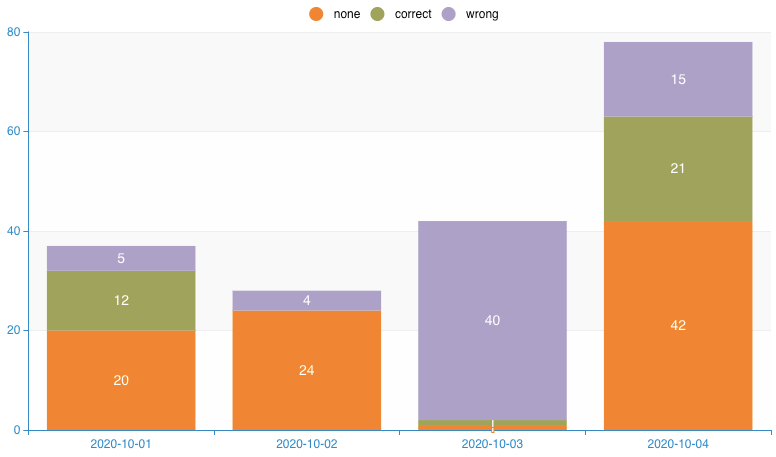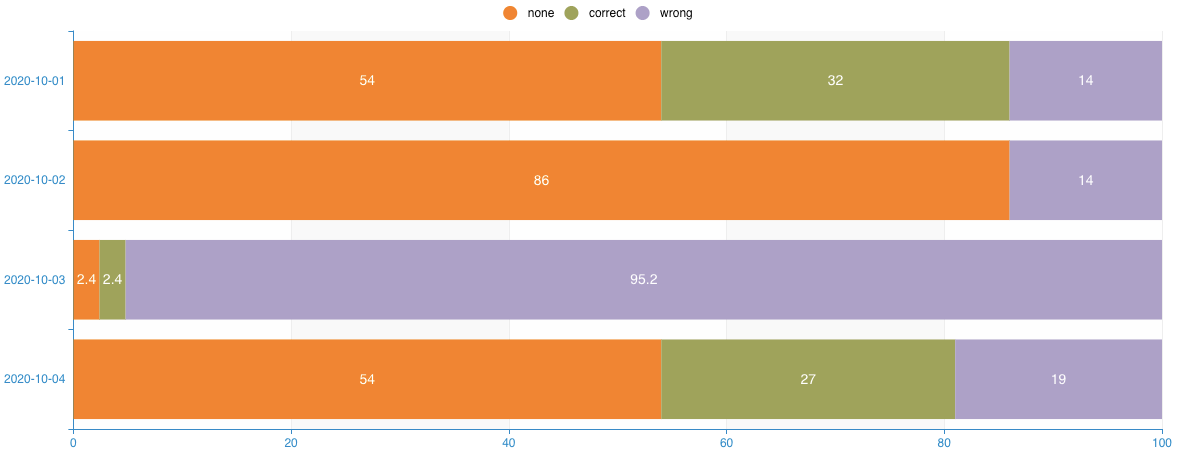# Stacked Bar, Horizontal Stacked Bar, and Normalized Horizontal Stacked Bar

## Introduction

The Stacked Bar Chart displays data of one series, separated into different categories ("stacks") as stacked bars:Stacked Bar Chart
When selecting Horizontal stacked Bar the same is displayed horizontally:Horizontal stacked Bar Chart
The Normalized horizontal stacked Bar Chart (also known as 100% stacked bar) shows the stacks' size as the proportioned amount of the total amount, i.e. the percentage of the stack's value based on the total value of all the stacks in the corresponding bar:Normalized horizontal stacked Bar Chart

## Expected Data and Graph Designer

All the variations of the Stacked Bar Charts expect data in a three column format, with the x-axis (for the (Normalized) Horizontal stacked Bar Chart: y-axis; the following mentions of x-axis are analogous for the y-axis for the (Normalized) Horizontal stacked Bar Chart) value as the first column, the stack name as the second column, and the value of the stack as the third column:
 Date Type Amount 2020-10-01 none 20 2020-10-01 correct 12 2020-10-01 wrong 5 2020-10-02 none 24 2020-10-02 wrong 4 2020-10-03 none 1
So there needs to be a row for every x-axis value and corresponding stack. If a stack does not have a value for a certain x-axis value it can be left out as shown in the example above (stack correct for x-axis value 2020-10-02).
Therefore, new stacks can simply be added by adding a new row with the stack's data for the corresponding x-axis value.
This required format can be achieved in SQL with a GROUP BY statement which groups by the x-axis value and the stack name and forms the sum of the stack value.
The selection of the x-axis in the Graph Designer does not have an effect.
Please make sure that all amounts are numbers and not strings. If your chart does not look as you expected, please cast the amount as integer as shown in this SQL query example:
SELECT Date, Type, CAST(amount as INT) as Amount FROM yourtable;